SBOS673D September   2017  – December 2018

PRODUCTION DATA.

1. Features
2. Applications
3. Description
4. Revision History
5. Pin Configuration and Functions
6. Specifications
7. Detailed Description
1. 7.1 Overview
2. 7.2 Functional Block Diagrams
3. 7.3 Feature Description
4. 7.4 Device Functional Modes
8. Application and Implementation
1. 8.1 Application Information
2. 8.2 Typical Applications
1. 8.2.1 Active Filters
2. 8.2.2 Implementing a 2:1 Active Multiplexer
3. 8.2.3 1-Bit PGA Operation
9. Power Supply Recommendations
10. 10Layout
11. 11Device and Documentation Support
12. 12Mechanical, Packaging, and Orderable Information

• DCK|5
• DBV|6
• DCK|5

### 6.8 Typical Characteristics: VS = 5.0 V

at VS+ = 5.0 V, VS– = 0 V, VOUT = 2 VPP, RF = 0 Ω, RL = 2 kΩ, G = 1 V/V, input and output referenced to mid-supply, and TA ≈ 25°C (unless otherwise noted)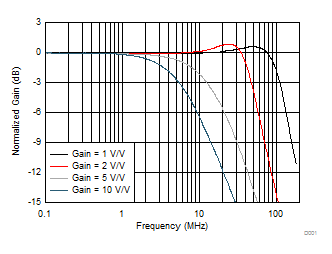See Figure 74 and Table 2, VOUT = 20 mVPP, RLOAD = 2 kΩ
Figure 1. Noninverting Small-Signal Frequency Response
vs Gain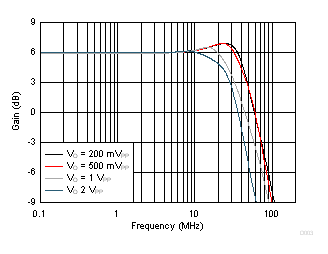Gain = 2 V/V, RLOAD = 2 kΩ
Figure 3. Noninverting Large-Signal Bandwidth vs VOPP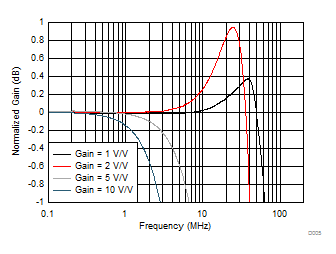See Figure 74 and Table 2, VOUT = 200 mVPP, RLOAD = 2 kΩ
Figure 5. Noninverting Response Flatness vs Gain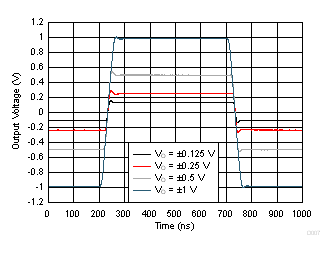See Figure 74, gain = 2 V/V, input edge rate set to stay below slew limiting
Figure 7. Noninverting Step Response vs Time and VOPP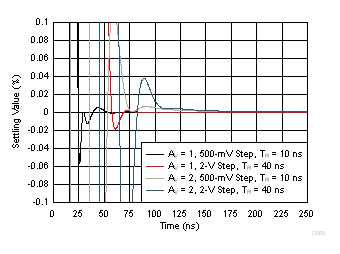See Figure 74 and Table 2
Figure 9. Simulated Noninverting Settling Time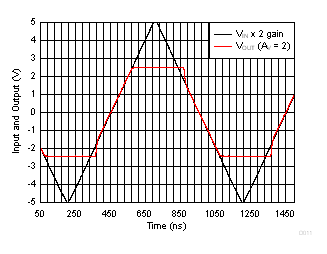See Figure 74 and Table 2, gain = 2 V/V
Figure 11. Noninverting Overdrive Recovery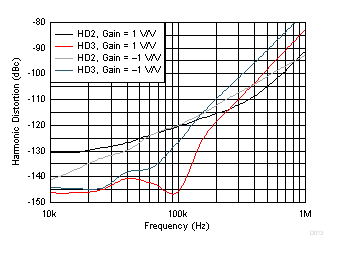See Figure 74, Figure 75, Table 2, and Table 3, VOUT = 2 VPP
Figure 13. Harmonic Distortion vs Frequency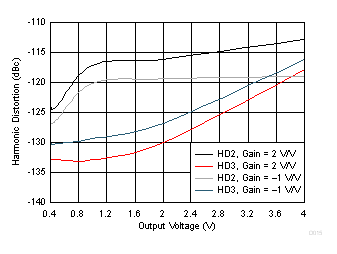See Figure 74, Figure 75, Table 2, and Table 3, VOUT = 2 VPP, f = 100 kHz
Figure 15. Harmonic Distortion vs Output Voltage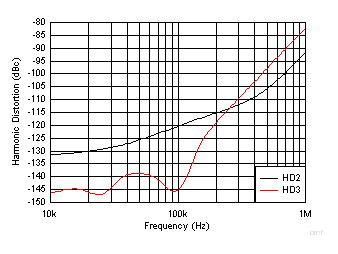See Figure 87, VOUT = 2 VPP, f = 100 kHz
Figure 17. Harmonic Distortion as Active Mux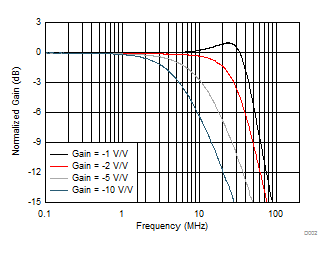See Figure 75 and Table 3, VOUT = 20 mVPP, RLOAD = 2 kΩ
Figure 2. Inverting Small-Signal Frequency Response
vs Gain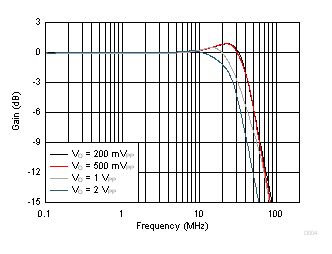Gain = –1 V/V, RLOAD = 2 kΩ
Figure 4. Inverting Large-Signal Bandwidth vs VOPP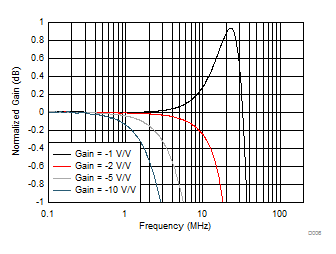See Figure 75 and Table 3, VOUT = 200 mVPP, RLOAD = 2 kΩ
Figure 6. Inverting Response Flatness vs Gain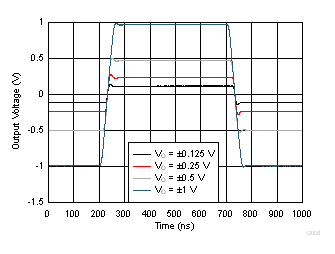See Figure 75, gain = –1 V/V, input edge rate set to stay below slew limiting
Figure 8. Inverting Step Response vs Time and VOPP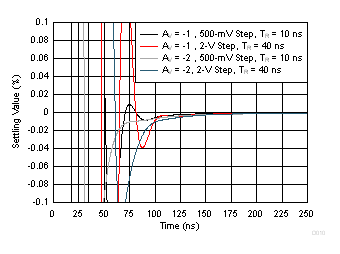See Figure 75 and Table 3
Figure 10. Simulated Inverting Settling Time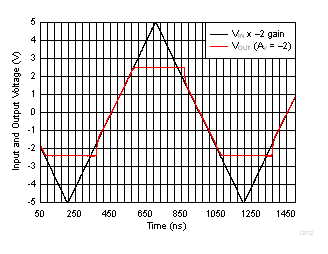See Figure 75 and Table 3, gain –2 V/V
Figure 12. Inverting Overdrive Recovery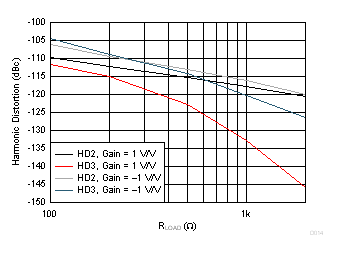See Figure 74, Figure 75, Table 2, and Table 3, VOUT = 2 VPP, f = 100 kHz
Figure 14. Harmonic Distortion vs RLOAD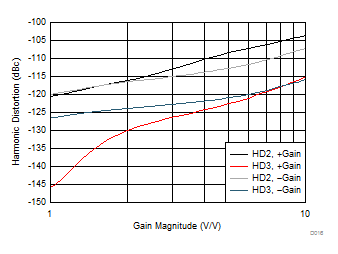See Figure 74, Figure 75, Table 2, and Table 3, VOUT = 2 VPP, f = 100 kHz
Figure 16. Harmonic Distortion vs Gain MagnitudeSee Figure 87, gain of 1 V/V or 2 V/V, VOUT = 2 VPP, f = 100 kHz
Figure 18. Harmonic Distortion as 1-Bit PGA